# Kirchhoff's Current and Voltage Laws

## Kirchhoff's Current Law and Nodal Analysis

Kirchhoff's Current Law (KCL) says that the current going into a junction or node is equal to the current going[[Image: out of a node. In other words, the sum of the currents entering the node must be zero (if we consider currents leaving the node to be a negative current entering the node). Consider the following diagram:

For the node A in the center, i1 and i2 are entering the node, and i3 and i4 are leaving the node. We would write: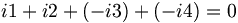$i1+i2+(-i3)+(-i4)=0\,$ which can also be written as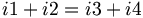$i1+i2=i3+i4\,$

Note that i7 is equal to i2; we can prove this by analyzing node B. We can also treat everything between node C and D as one big node, and conclude that i5 is equal to i6 without having to know the value of any of the currents within.

When solving for the currents in a real problem, we can choose arbitrarily in which direction the arrows point. If we guessed incorrectly, the value we obtain after solving for the current will be negative. However, if you draw i4 as leaving node A as in the diagram above, don't draw i4 as leaving node D when you are writing your node equations.

]]If we had drawn the currents for node A as following:

Then our node equation looks like:$i1+i2+i3+i4=0\,$

Unless all the currents are zero, one or more of the currents must turn out to be negative. The negative currents will flow in the direction opposite from that which the arrow is pointing.

## Kirchhoff's Voltage Law and Loop Analysis

Kirchhoff's Voltage Law (KVL) states that the sum of the voltages around any closed loop is equal to zero. Also, the voltage between any two nodes is the same no matter which path is taken. In the following diagram, it doesn't matter which path you choose(or which direction you go in) to add the voltages of the components from point A back to point A, they all must add up to zero.

Also, the voltage between any two points does not depend on which path you take. In the following diagram, the voltage between point A and point B is the same no matter which path is chosen.

To keep all the positive and negative signs lined up when solving a circuit, we use the following sign convention for the voltage and current for the components:

If the current flowing in the direction of the arrow, then the power absorbed by the component is p = iv, and the component absorbs a positive amount of power. If the current is flowing out of the positive terminal, we can assign a negative current or negative voltage to the component in order to preserve our sign convention. Then, the power absorbed p = iv will come out to be negative. This means that the component is actually supplying power. Note that resistors cannot store power (and hence cannot supply power); the energy absorbed by a resistor is lost as heat energy.

The circuit below shows a battery, resistor, inductor, and capacitor connected in series.

In this circuit, we have chosen to draw the current flowing clockwise and assign the voltages for our components according to our sign convents. We then add up the voltages of the components, following the loop. It doesn't matter if you go clockwise or counter-clockwise. If the signs across a component goes from positive to negative (in the direction of your loop), this indicates a drop in voltage; if it goes from negative to positive, this indicates a rise in voltage.

In the example above, if we choose to start in the bottom left corner and move in the clockwise direction, our equation looks like: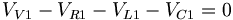$V_{V1}-V_{R1}-V_{L1}-V_{C1}=0\,$

or if we go in the counter-clockwise direction, we get: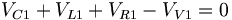$V_{C1}+V_{L1}+V_{R1}-V_{V1}=0\,$

which is the same thing.

If we had drawn the current going in a counter-clockwise direction, our circuit diagram would look like this: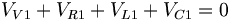$V_{V1}+V_{R1}+V_{L1}+V_{C1}=0\,$

If we know that VV1 is positive, then VR1 + VL1 + VC1 will turn out to be negative, and we know we have our signs flipped.

## Example and Practice Problems

### Example 1

Find the voltage and current of each resistor at the steady state of the system.

Answer: At steady state, the capacitor becomes charged and acts like an open circuit, and the inductor acts like a short circuit. Therefore, at steady state, our system is equivalent to:

First, simplify the network of resistors. The combined resistance of the three resistors is equal to: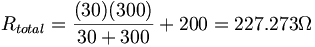$R_{total}=\frac{(30)(300)}{30+300}+200=227.273\Omega$

The voltage across the resistors is 10V, and using ohm's law, we find that the current going through the circuit is I = 10 / 227.273 = 0.044A. This is the current flowing through the voltage source R1. Kirchhoff's voltage law tells us that the voltage or R2 and R3 are the same, and the sum of R1 and R2 is equal to 10V. We can find the voltage of R1 by using Ohm's law again: V = (0.044A)(200Ω) = 8.8V. Then R2 and R3 must have 10 − 8.8 = 1.2V across them, and from that we can calculate that R2 's current is I = 1.2 / 30 = 0.04A, and R3 's current is I = 1.2 / 300 = 0.004A. Note that the sum of the currents for R2 and R3 is equal to the current in R1.

### Example 2

Find the voltage and current of each element in the system.

We will use loop analysis to solve this circuit:

We can solve this by writing two loop equations using KVL--one for each loop. Note that R3 and V1 have both i1 and i2 going through them; therefore, the total currents going through R3 and V1 will be the superposition of i1 and i2.

For the loop on the left (in the direction of the current):

0 = VV1VR3VR1

0 = V1 − (i1i2)R3 − VR1

0 = 10 − 1000(i1i2) − 1000(i1),

and for the loop on the right:

0 = VV2VR2VR3V1

0 = VV2i2R2 − (i2i1)R3VV1

0 = 20 − 2000i2 − 1000(i2i1) − 10

Now we have two equations and two unknowns, which is easily solved:

i1 = 8mA and i2 = 6mA

Now we use ohm's law and calculate our voltages:

VV1 = 10V

VV2 = 20V

VR1 = 8V

VR2 = 12V

VR3 = 2V

And our currents:

IV1 = 6mA

IV2 = 2mA

IR1 = 8mA

IR2 = 6mA

IR3 = 2mA

Note that we could also have drawn our loops like this:

In this case, our equations would be:

for the small loop:

0 = VV1VR3VR1

0 = 10 − 1000(i1) − 1000(i1 + i2)

and for the big loop:

0 = VV2VR2VR3

0 = 20 − 2000i2 − 1000(i2 + i1)

Solving for i1 and i2, we find that:

i1 = 2mA

and

i2 = 6mA

The current going through R1 is equal to i1 + i2 = 8mA, which matches our previous answer.

### Practice Problem 1

Solve for the voltage and current in each of the components:

### Practice Problem 2

Solve for the voltage and current in each of the resistors at time$t=0\,$ and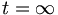$t=\infty$. Assume that both the inductor and the capacitor are uncharged in their initial states.